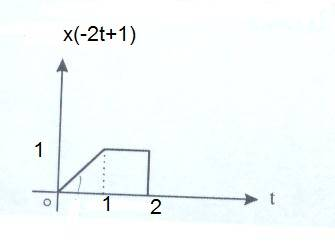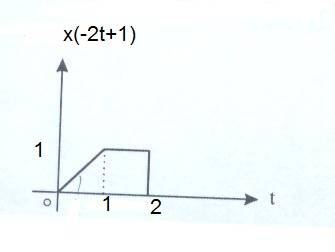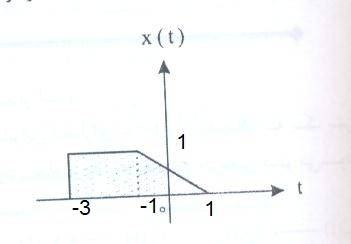# Find x(t) from a x(-2t+1)

baby_1
Hello
here is the x(-2t+1). i want to know how can find the x(t) diagram from this picture?Mentor
Take t=1 for example: You know that 1=x(-2*1+1)=x(-1).
Does that help?

Hello
here is the x(-2t+1). i want to know how can find the x(t) diagram from this picture?Hi baby_1, try taking it in baby stepsOne simple transformation at a time.

- See if you can deduce what x(-2t) would be. Hint x(-2t+1) = x(-2(t-0.5)).

- From there you can probably figure out x(2t).

- From there you can try to deduce what x(t) will look like.

Last edited:
baby_1
Dear mfb
Thanks it helps me to solve many problems